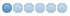• lagore
• Offline
• New Member
•02 Nov 2018 20:58 #119849 by lagore
There are two errors in the thread milling routine,
The first is in the "(convert size to machine units)" subroutine it specifies "if [#<units> EQ 1] (inch threads)" but in the config file (thread-milling.cfg) units = 1 is metric therefore the metric size is multiplied by 25.4 giving the incorrect size.
The other error is when cutting the partial turn lefthand thread and the implementation of 'polar coordinates' in gcode. Polar coordinates start angle is at "X positive" (3 o'clock) and move in an anticlockwise direction, a righthand thread is cut with a G3 (anticlockwise) so therefore the two operate correctly e.g. a 3/4 turn is a 270° move specifying the endpoint in polar coordinates which will be 6 o'clock, with an anticlockwise move from 3 o'clock to 6 o'clock you get 270° but with a lefthand thread it is a G2 (clockwise move) from 3 o'clock to 6 o'clock a 90° turn. To summerise for a lefthand thread you will get 360 - <partial_turn_angle) for the move. For turns >180° you get the pitch cut for too short of a turn and vise versa.
The following user(s) said Thank You: FernV